# Linear Programming Relaxation

The Linear Programming (LP) relaxation is the same as the original integer programme, except we "relax" the integer variables to allow them to take fractional values. The integer programme's feasible region lies within the feasible region of the LP relaxation (at points where the integer variables have integer values). Therefore the integer restrictions cause the optimal objective function value to be worse in the integer programme as compared to the LP relaxation. However, if a solution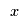of the LP relaxation has integer values for the integer variables, thenalso solves the integer programme. In some cases, if the solution values for the integer variables are large, then rounding the LP relaxation solution may give a good solution to the integer programme. However, you need to make sure that the rounded solution is not infeasible!

For some classes of problem the LP relaxation gives naturally integer solutions:

• An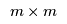matrix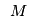is unimodular if and only if its determinant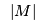is equal to 1 or –1;
• An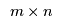matrixis totally unimodular if and only if everynon-singular submatrix ofis unimodular;
• If the constraint matrix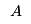and the right-hand side vector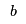of a mixed-integer programme are totally unimodular and integer respectively, then the mixed-integer programme is naturally integer and the LP relaxation solution is the optimal solution *The transportation problem is an example of one such problem;
• Most network flow problems are also naturally integer;
• Some scheduling problems are naturally integer.

## Using AMPL

In AMPL the LP relaxation may be solved simply by turning on the relax_integrality option:

option relax_integrality 1;


Turning it off:

option relax_integrality 0;